### Tribute to LittleViking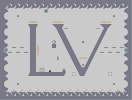Hover over the thumbnail for a full-size version.

Author bigblargh action author:bigblargh playable unrated 2005-12-20 2 more votes required for a rating. \$Tribute to LittleViking#bigblargh#none#=:96=:96=6=0:9:96=:96==:000000000000000000000:=000000000000000000000=:000000000000000000000:=000000000000000000000=:000000000000000000000:=0000LOOOOOOOOOOOK0000=:000011111111111110000:=0000I0000000000010000=:000000000000000010000:=000000000000000010000=:000000000000000010000:=000040000100000010000=:00001LH00000000010000:=0000MJ1LH000000G10000=:000000FJ1LH0000FI0000:=00000000FJ1LH00000000=:0000000000FJ1LH000000:=000000000000FJ1LH0000=:00000000000000F110000:=0000000000000GKMI0000=:00000000000GKMI000000:=000000000GKMI00000000=:0000000GKMI0000000000:=00004GKMI000000000000=:00001MI00000000000000:=000050000000000000000=:000000000000000000000:=000000000000000000000=:000000000000000000000:=;87<;87<7<0;8;87<;87<=|5^348,348!8^36,300,0!8^756,300,2!0^180,132!0^192,132!0^204,132!0^216,132!0^324,132!0^336,132!0^348,132!0^360,132!0^372,132!0^612,132!0^624,132!0^636,132!0^648,132!0^660,132!10^498,252!11^252,420,498,390!0^708,540!0^660,540!0^612,540!0^564,540!0^516,540!0^468,540!0^420,540!0^372,540!0^324,540!0^276,540!0^228,540!0^180,540!0^132,540!0^84,540!12^312,552!12^360,552!12^408,552!12^456,552!12^504,552!12^552,552!12^600,552!12^648,552!12^696,552!12^744,552!12^168,552!12^120,552!12^72,552!12^48,48!12^96,48!12^144,48!12^192,48!12^240,48!12^288,48!12^336,48!12^384,48!12^432,48!12^480,48!12^528,48!12^576,48!12^624,48!12^672,48!12^720,48!3^108,504!3^684,504!3^684,96!3^108,96!9^60,312,1,0,2,13,0,0,-1!9^108,312,1,0,4,13,0,0,-1!9^156,312,1,0,6,13,0,0,-1!9^132,288,1,0,5,11,0,0,0!9^84,288,1,0,3,11,0,0,0!9^732,288,1,0,30,11,0,0,0!9^684,288,1,0,28,11,0,0,0!9^636,288,1,0,26,11,0,0,0!9^612,312,1,0,25,13,0,0,-1!9^660,312,1,0,27,13,0,0,-1!9^708,312,1,0,29,13,0,0,-1!1^444,444!1^660,444!4^300,426,1!8^276,66,1!0^228,324!0^228,312!0^228,300!0^228,288!0^228,276!0^480,348!0^492,348!0^504,348!0^516,348!2^714,552,0,-1!2^90,552,0,-1!12^216,552!12^264,552!2^498,408,0,-1!6^324,252,0,0,1,0!7^588,180,3!7^396,180,3# This is a tribute to LV, because he correctly identified the type of verbal that "boring" is in this sentence: This conversation is boring. If anyone all-golds this, I'll make 'em a ded. Because I'm bored.

## Other maps by this author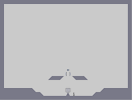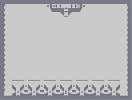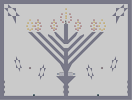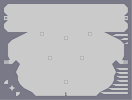Head of a pin Nausea Inner n00b IB|\ Menorah Un-Metanet